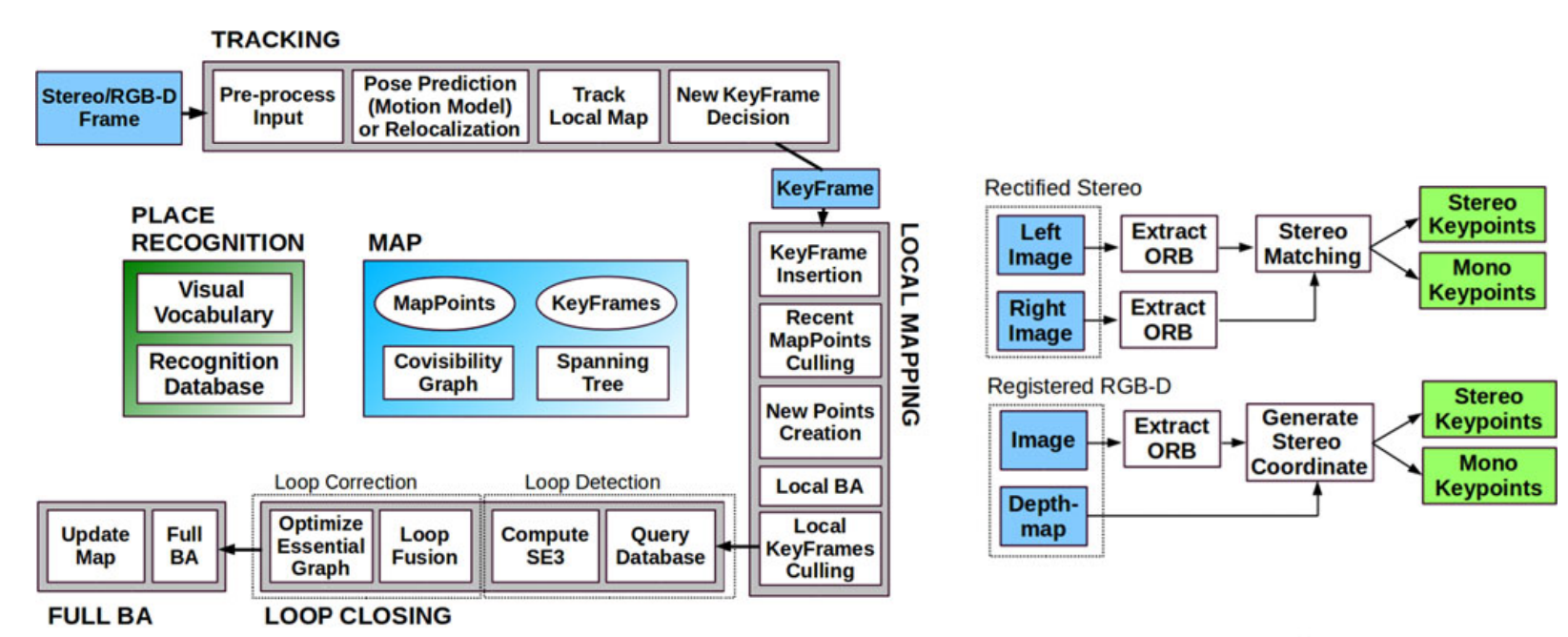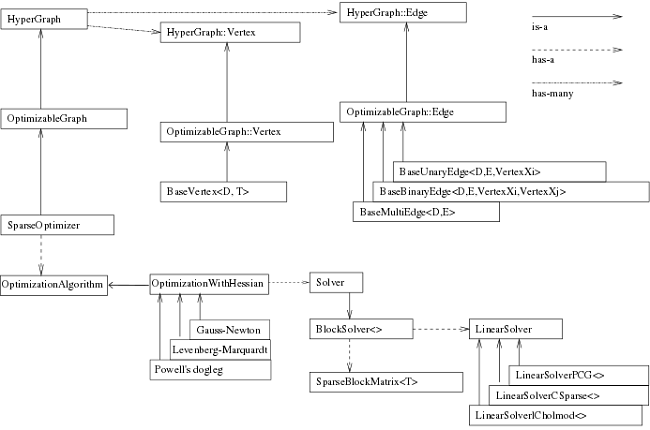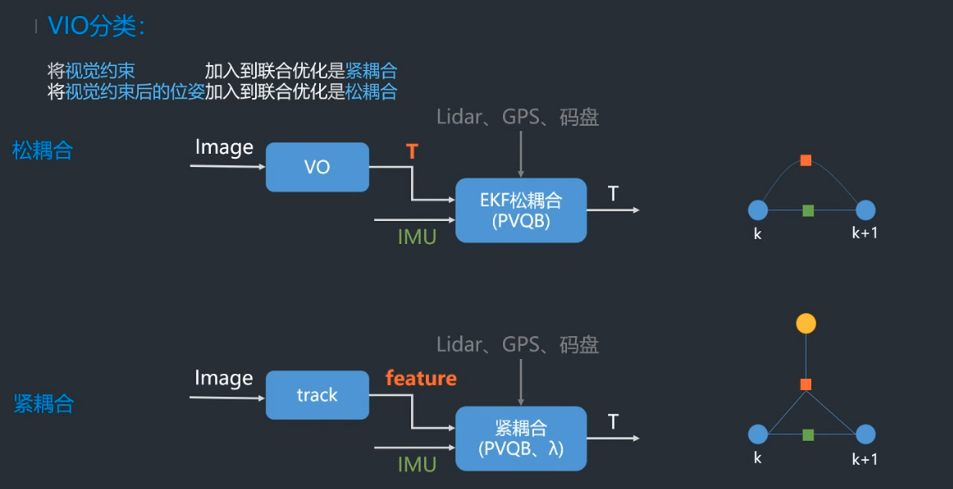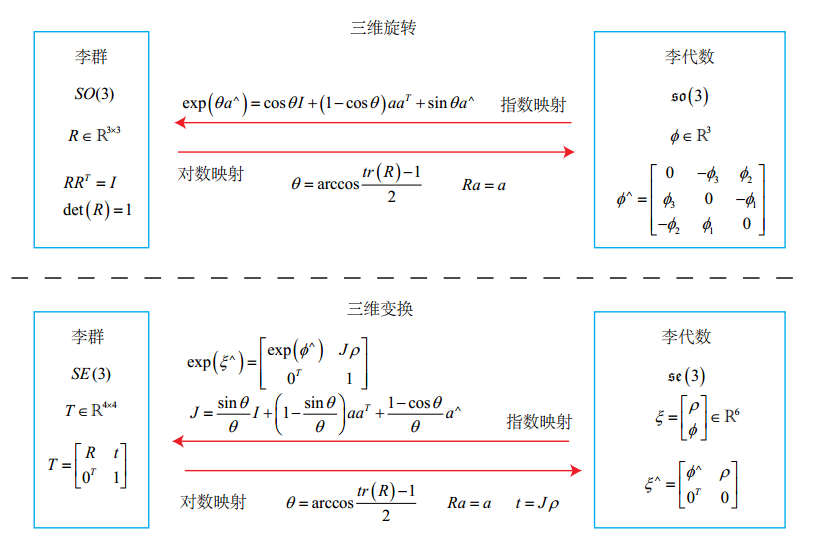• 视觉SLAM 视觉SLAM 视觉SLAM 视觉SLAM 视觉SLAM 视觉SLAM
• 视觉SLAM知识点视觉传感器的分类单目相机双目相机RGBD视觉特征点SLAM计算流图噪声处理 没什么好说的，最近在ROS下做视觉计算。双目摄像机导航。推荐高翔的《视觉SLAM十四讲》。知识点很全，推导也很详细。随便写一下...
视觉SLAM知识点视觉传感器的分类单目相机双目相机RGBD视觉特征点SLAM计算流图噪声处理
没什么好说的，最近在ROS下做视觉计算。双目摄像机导航。推荐高翔的《视觉SLAM十四讲》。知识点很全，推导也很详细。随便写一下吧，但项目压在手里，很烦。想起来就写一点笔记。
视觉传感器的分类
视觉传感器分为主要的三种吧。单目相机（Monocular），双目摄像机（stereo），单目加红外（RGBD）。
单目相机
单目相机便宜。但反解决自身姿态会很麻烦。一般用对极几何计算摄像的旋转矩阵R，平移矩阵T。通过三角计算可以计算得到。但这里就有一个问题。在相机纯旋转下，无法计算。所以你在跑单目相机的slam时，初始化第一帧图像一定需要平移一下摄像头。单目相机很多，随便一个网络相机就可做
双目相机
双目相机，emmm。我觉得是一个坑。但很符合人类认知世界的方式。人通过双眼视差理解物体远近，不可能精确获得物体深度信息。但是我只能说这个会比RGBD坑一点。因为视差图很消耗计算资源。而且获得深度图用可能穿过实际物体。这是有像素误匹配导致深度计算过大。双目相机，zed，小觅相机（mynteye）
RGBD
采用RGB相机和红外结构光计算物体深度和获取图像。由于红外结构光通过物理方法计算深度，是不会穿过墙面，但任何传感器实际使用都会存在噪声。kinect或其他3D体感相机都可。
视觉特征点
常用视觉特征点计算SIFT，SUFT。

检测区域
SIFT
SURF

尺度空间
DOG与不同尺度的图片卷积
不同尺度的box filters与原图片卷积

特征点检测
先进行非极大抑制，再去除低对比度的点。再通过Hessian矩阵去除边缘的点
先利用Hessian矩阵确定候选点，然后进行非极大抑制

特征方向
在正方形区域内统计梯度的幅值的直方图，找max对应的方向。可以有多个方向。
在圆形区域内，计算各个扇形范围内x、y方向的haar小波响应，找模最大的扇形方向

特征描述子
采样点划分，计算每个区域的采样点的梯度方向和幅值，统计成直方图，一共128维
2020的区域划分为44的子区域，每个子区域找5*5个采样点，计算采样点的haar小波响应，记录∑dx,∑dy,∑

变种的算法很多，不介绍了。原因就是慢。
采用的是ORB。FAST特征点获取和bried二进制特征描述子。
ORB-SLAM: a versatile and accurate monocular SLAM system
ORB-SLAM2 an open-source SLAM System for Monocular, Stereo and RGB-D Camera
这个系统很不错。
ORB速度很快，FAST通过圆形区域内像素灰度查找，可以快速查到角点。由于只是查找到角点，角点不具备旋转不变性。通过计算两方向的灰度变化获得角度值，从而定义一个图像矩。可以描述灰度变化方向。
SLAM计算流图使用三个线程，主要计算特征点并决定是否加入关键帧。局部关键帧匹配。回环检测（检测是否观察相同区域，如果观察到相同的特征会匹配之前观测帧并更新全部关键帧数据）
噪声处理
只要是个传感器就会引入噪声。滤波是常用算法，卡尔曼滤波及其变种。Extended Kalman filter引入非线性优化，非线性优化在做匹配时非常重要。比如高斯牛顿法，Levenburg-Marquart这类采用二阶矩匹配，效果都很好。由于采用非线性，也有研究者将深度学习引入SLAM匹配，方法很多，效果不一。kitti数据集里有效果比对。另一种是开源图优化，21世纪最大发现就是在机器终端维护的二阶矩是稀疏的。图优化采用特殊方法快速计算。原理我还没搞明白。嗯，加油吧，希望项目能做完。


展开全文• 转自http://blog.csdn.net/qq_18661939/article/details/51782376（1) orb_slam 官网（网站最后有5篇论文，价值很高）http://webdiis.unizar.es/~raulmur/orbslam/（2)半仙居士blog（可以都看，很经典）...
转自http://blog.csdn.net/qq_18661939/article/details/51782376（1) orb_slam 官网（网站最后有5篇论文，价值很高）http://webdiis.unizar.es/~raulmur/orbslam/（2)半仙居士blog（可以都看，很经典）http://www.cnblogs.com/gaoxiang12/（3) 贺一加 blog（monocular slam 和navigation讲的很好可以看看）http://blog.csdn.net/heyijia0327（4)开源代码汇总openslam （里面几乎有所有开源代码）             https://www.openslam.org/ （5)slam视频教程http://pan.baidu.com/s/1o6Oku4y 密码:sd4c（6)书籍Probabilistic Robotics     链接:http://pan.baidu.com/s/1o6MOiJw 密码:iqcfMultiple View Geometry in Computer Vision Second Edition   Robotics Vision and Control （7)PCL官网（里面的教程都可以看看，比较简单）http://pointclouds.org/documentation/tutorials/（8)opencv学习（很详细）http://blog.csdn.net/column/details/opencv-manual.html（9)opencv视频http://pan.baidu.com/s/1i37nXSL 密码: 3xnd    （10) ros学习（机器人实物开发所必须的，里面好多开源code，好多教程，好多有用的插件，总之特别好）http://wiki.ros.org/cn/（中文版）http://wiki.ros.org/（英文版）（11)视觉做的很好的网站computer vision group（lsdslam就是他们做的）http://vision.in.tum.de/research （12)一个slam资料介bloghttp://blog.csdn.net/akunainiannian/article/details/45363731  （13)orbslam论文翻译（翻译的不错）http://blog.csdn.net/cicibabe/article/details/50631431http://www.360doc.com/content/16/0512/17/478627_558566052.shtml（14)不错的bloghttp://www.qiqitek.com/blog.html(15)冯兵的bloghttp://www.fengbing.net(16)ros 很好的教程http://dscl.lcsr.jhu.edu/ME530707_2014(17)orbslam2  Androidhttps://github.com/FangGet/ORB_SLAM2_Android(18)orbslam2 maphttps://github.com/MathewDenny/ORB_SLAM2(19) orbslam code 讲解http://www.cnblogs.com/luyb/p/5260785.html(20) 一篇VO外文bloghttp://avisingh599.github.io/blog/（21)orbslam2代码解析http://git.oschina.net/paopaoslam/ORB-SLAM2（22）imu和单目的数据融合开源代码（EKF)https://github.com/ethz-asl/rovio（23）imu和单目的数据融合开源代码https://github.com/ethz-asl/okvis_ros(非线性优化）（24)解决初始化很慢的问题https://github.com/poine/ORB_SLAM2（25)解决点云mesh和建立网格map的c++库http://www.cgal.org/（26）orbslam+imu（立体相机）https://github.com/JzHuai0108/ORB_SLAM（27）微信公众号（里面一些大神的讲解）泡泡机器人(28) 百度文库一篇讲解双目视觉原理的PPThttp://wenku.baidu.com/link?url=sYrfx0EX9DVDOXlVngjRQuYDQHsXQ16ScMr3CnkjJ-0b1-BlL9tVqkhmawF4bFYcHXBiY3uAO2Re1_SWndi1PzapxwMQQwLeAI7VOMcGRrW(29)google open sourcehttps://github.com/googlecartographer（30)高翔的slambook（很基础，很适合代码学习）https://github.com/gaoxiang12/slambook(31)计算机视觉的一些库文件http://blog.csdn.net/garfielder007/article/details/50533052http://blog.csdn.net/dan1900/article/details/41726507一，入门篇1. Andrew Davison的课程： http://www.doc.ic.ac.uk/~ajd/Robotics/index.html    AD在在week 8里面推荐了slam的两个入门 Tutorial 1  和Tutorial 22. Tutorial的两篇文章文笔灰常秀丽，但是不操作还是云里雾里：   所以这里有一个瑞士苏黎世理工的学生练习   大家把excise 3：SLAM（EKF）做了，也就差不多了解些slam的原理了   关于练习3的答案，我过几天上传好, 答案3. 对于我这个学渣来说，EKF其实还是比较难理解，所以推荐一本书，详见第三章，学霸无视二、现有资源1. OpenSLAM：https://openslam.org/这个网站中含有很多slam方面的资料，编写的程序也各有不同，很权威2. Kitti这个图库，大家可以下载做simulation：http://www.cvlibs.net/datasets/kitti/3. 个人感觉exercise 3练习后，可以选择 Javier Civera 的程序进行试手，感觉灰常不错。注意对calibration的调整    http://webdiis.unizar.es/~jcivera/code/1p-ransac-ekf-monoslam.html4. 对于JC的1p RANSAC-monoSLAM有一定了解了，可以试试用SURF去实现    这里有个南理工哥们的论文还不错，可以参考 http://cdmd.cnki.com.cn/Article/CDMD-10288-1012319519.htm三、有用的书籍1. Multiple View Geometry in Computer Vision Second Edition ，http://www.robots.ox.ac.uk/~vgg/hzbook/    计算机视觉方面大神级别的书，也有中文版，点此下载中英文双版2. Robotics Vision and Control  ， pdf下载：http://robotics.itee.uq.edu.au/~metr4202/tpl/Robotics%20Vision%20&%20Control.pdf通过MATLAB几乎把机器人学给贯穿了，里面每章节都有对应的Code，关于里面Matlab的codes，需要留言澳大利亚昆士兰理工大学的Peter Corke是机器视觉领域的大牛人物，他所编写的Robotics, vision and control一书更是该领域的经典教材配套有matlab工具箱。工具箱分为两部分，一部分为机器人方面的，另一部分为视觉方面的工具箱源代码都是开放免费下载的： http://petercorke.com/Toolbox_software.html3. Probabilistic Robotics. 这本书是导师推荐的他说这本书的理解要有很好的数学基础，大神一定要读，很多不懂的都会柳暗花明http://www.probabilistic-robotics.org/1. http://www.openslam.org/ 各种实现的SLAM源码。2. http://cres.usc.edu/radishrepository/view-all.php 一大波dataset3. http://www-personal.acfr.usyd.edu.au/nebot/victoria_park.htm 4. Ronald Parr; (DP-SLAM创始者,从文章到数据,程序都公开的牛人)知乎大神讨论：https://www.zhihu.com/question/35186064
展开全文• 视觉SLAM面试题汇总视觉SLAM面试题汇总1. SIFT和SUFT的区别2. 相似变换、仿射变换、射影变换的区别3. Homography、Essential和Fundamental Matrix的区别4. 视差与深度的关系5. 描述PnP算法6. 闭环检测常用方法7. 给...
视觉SLAM总结——视觉SLAM面试题汇总视觉SLAM总结——视觉SLAM面试题汇总1. SIFT和SUFT的区别2. 相似变换、仿射变换、射影变换的区别3. Homography、Essential和Fundamental Matrix的区别4. 视差与深度的关系5. 描述PnP算法6. 闭环检测常用方法7. 给一个二值图，求最大连通域8. 梯度下降法、牛顿法、高斯-牛顿法的区别9. 推导一下卡尔曼滤波、描述下粒子滤波10. 如何求解$Ax=b$的问题11. 什么是极线约束12. 单目视觉SLAM中尺寸漂移是怎么产生的13. 解释SLAM中的绑架问题14. 描述特征点法和直接法的优缺点15. EKF和BA的区别16. 边缘检测算子有哪些？17. 简单实现cv::Mat()18.  10个相机同时看到100个路标点，问BA优化的雅克比矩阵多少维19. 介绍经典的视觉SLAM框架20. 介绍下你熟悉的非线性优化库21.室内SLAM与自动驾驶SLAM有什么区别？22. 什么是紧耦合、松耦合？优缺点。23. 地图点的构建方法有哪些24. 如果对于一个3D点，我们在连续帧之间形成了2D特征点之间的匹配，但是这个匹配中可能存在错误的匹配。请问你如何去构建3D点？25. RANSAC在选择最佳模型的时候用的判断准则是什么?26. 除了RANSAC之外，还有什么鲁棒估计的方法？27. 3D地图点是怎么存储的？表达方式？28. 给你m相机n个点的bundle adjustment。当我们在仿真的时候，在迭代的时候，相机的位姿会很快的接近真值。而地图点却不能很快的收敛这是为什么呢？29. LM算法里面那个 $\lambda$ 是如何变化的呢？30. 说一下3D空间的位姿如何去表达?31. 李群和李代数的关系32. 求导$\frac{\partial R_{1} R_{2}}{\partial R_{1}}$33. Mat是如何访问元素的？先访问行还是先访问列？34. 写出单目相机的投影模型，畸变模型。35. 安装2D lidar的平台匀速旋转的时候，去激光数据畸变，写代码35. 给两组已经匹配好的3D点，计算相对位姿变换，写代码36. ORB-SLAM初始化的时候为什么要同时计算H矩阵和F矩阵？37. 说一下Dog-Leg算法38. Vins-Mono里面什么是边缘化？First Estimate Jacobian？一致性？可观性？39. 说一下VINS-Mono的优缺点40. 推导一下VINS-Mono里面的预积分公式41. 在给定一些有噪声的GPS信号的时候如何去精准的定位？42. 如何标定IMU与相机之间的外参数？43. 给你xx误差的GPS，给你xx误差的惯导你怎么得到一个cm级别的地图?44. 计算H矩阵和F矩阵的时候有什么技巧呢？45. 给一组点云，从中提取平面。46. 给一张图片，知道相机与地面之间的相对关系，计算出图的俯视图。47. 双线性插值如何去做，写公式。48. RGB-D的SLAM和RGB的SLAM有什么区别？49 什么是ORB特征? ORB特征的旋转不变性是如何做的? BRIEF算子是怎么提取的?50 ORB-SLAM中的特征是如何提取的？如何均匀化的？参考个人面经51. 为什么射影变换自由度要减一？52. 解释下EPnP53. Lab色域是什么？54. 解释下并查集的结构55. Canny算子的流程？57. 简单说下其他边缘检测算子58. SLAM中采用逆深度的原因？59. SFM和SLAM里面的BA有什么区别？60. ORB SLAM里面的Local Map和VINS-mono里面的滑窗法有什么区别？61. RPE、ATE和RMSE分别是什么？62. ORB SLAM有什么缺点？
视觉SLAM总结——视觉SLAM面试题汇总
秋招基本上已经结束了，已经签到了自己向往公司的offer，下面是我准备秋招过程中从网上搜集的以及自己面试过程中遇到的一些和视觉SLAM相关的面试题，秋招不易，自己从前辈的分享中收获了很多，我也是希望通过自己的分享帮助到更多的人。
1. SIFT和SUFT的区别

构建图像金字塔，SIFT特征利用不同尺寸的图像与高斯差分滤波器卷积；SURF特征利用原图片与不同尺寸的方框滤波器卷积。
特征描述子，SIFT特征有4×4×8=128维描述子，SURF特征有4×4×4=64维描述子
特征点检测方法，SIFT特征先进行非极大抑制，再去除低对比度的点，再通过Hessian矩阵去除边缘响应过大的点；SURF特征先利用Hessian矩阵确定候选点，然后进行非极大抑制
特征点主方向，SIFT特征在正方形区域内统计梯度幅值的直方图，直方图最大值对应主方向，可以有多个主方向；SURF特征在圆形区域内计算各个扇形范围内x、y方向的haar小波响应，模最大的扇形方向作为主方向

2. 相似变换、仿射变换、射影变换的区别
等距变换：相当于是平移变换（t）和旋转变换（R）的复合，等距变换前后长度，面积，线线之间的角度都不变。自由度为6（3+3）
相似变换：等距变换和均匀缩放（S）的一个复合，类似相似三角形，体积比不变。自由度为7（6+1）
仿射变换：一个平移变换（t）和一个非均匀变换（A）的复合，A是可逆矩阵，并不要求是正交矩阵，仿射变换的不变量是:平行线，平行线的长度的比例，面积的比例。自由度为12（9+3）
射影变换：当图像中的点的齐次坐标的一般非奇异线性变换，射影变换就是把理想点（平行直线在无穷远处相交）变换到图像上，射影变换的不变量是:重合关系、长度的交比。自由度为15（16-1）
参考：·多视图几何总结——等距变换、相似变换、仿射变换和射影变换
3. Homography、Essential和Fundamental Matrix的区别
Homography Matrix可以将一个二维射影空间的点变换该另一个二维射影空间的点，如下图所示，在不加任何限制的情况下，仅仅考虑二维射影空间中的变换，一个单应矩阵$H$可由9个参数确定，减去scale的一个自由度，自由度为8。Fundamental Matrix对两幅图像中任何一对对应点$\bm x$和$\bm x'$基础矩阵$\bm F$都满足条件：$\bm{x^T F x' = 0}$，秩只有2，因此F的自由度为7。它自由度比本质矩阵多的原因是多了两个内参矩阵。
Essential matrix：本质矩是归一化图像坐标下的基本矩阵的特殊形式，其参数由运动的位姿决定，与相机内参无关，其自由度为6，考虑scale的话自由度为5。
参考多视图几何总结——基础矩阵、本质矩阵和单应矩阵的自由度分析
4. 视差与深度的关系
在相机完成校正后，则有 $d/b=f/z$,其中 $d$ 表示视差，$b$ 表示基线，$f$ 是焦距，$z$ 是深度。这个公式其实很好记，在深度和焦距确定的情况下，基线越大，视差也会越大。5. 描述PnP算法
已知空间点世界坐标系坐标和其像素投影，公式如下目前一共有两种解法，直接线性变换方法（一对点能够构造两个线性约束，因此12个自由度一共需要6对匹配点），另外一种就是非线性优化的方法，假设空间坐标点准确，根据最小重投影误差优化相机位姿。
目前有两个主要场景场景，其一是求解相机相对于某2维图像/3维物体的位姿；其二就是SLAM算法中估计相机位姿时通常需要PnP给出相机初始位姿。
在场景1中，我们通常输入的是物体在世界坐标系下的3D点以及这些3D点在图像上投影的2D点，因此求得的是相机坐标系相对于世界坐标系(Twc)的位姿
在场景2中，通常输入的是上一帧中的3D点（在上一帧的相机坐标系下表示的点）和这些3D点在当前帧中的投影得到的2D点，所以它求得的是当前帧相对于上一帧的位姿变换
6. 闭环检测常用方法
ORB SLAM中采用的是词袋模型进行闭环检测筛选出候选帧，再通过求解Sim3判断最合适的关键帧
LSD SLAM中的闭环检测主要是根据视差、关键帧连接关系，找出候选帧，然后对每个候选帧和测试的关键帧之间进行双向Sim3跟踪，如果求解出的两个李代数满足马氏距离在一定范围内，则认为是闭环成功
7. 给一个二值图，求最大连通域
这个之后单独写一篇博客来研究这个好了，二值图的连通域应该是用基于图论的深度优先或者广度优先的方法，后来还接触过基于图的分割方法，采用的是并查集的数据结构，之后再作细致对比研究。
8. 梯度下降法、牛顿法、高斯-牛顿法的区别
在BA优化、PnP、直接法里面都有接触到非线性优化问题，上面几种方法都是针对对非线性优化问题提出的方法，将非线性最优化问题作如下展开，就可以获得梯度下降法和牛顿法梯度下降法是一个一阶最优化算法，通常也称为最速下降法。 要使用梯度下降法找到一个函数的局部极小值，必须向函数上当前点对应梯度（或者是近似梯度）的反方向的规定步长距离点进行迭代搜索。因此指保留一阶梯度信息。缺点是过于贪心，容易走出锯齿路线。牛顿法是一个二阶最优化算法，基本思想是利用迭代点处的一阶导数(梯度)和二阶导数(Hessen矩阵)对目标函数进行二次函数近似。因此保留二阶梯度信息。缺点是需要计算$\bm H$矩阵，计算量太大。
$\Delta \bm x^* = -\bm {J^T}(\bm x)/\bm H$
而把非线性问题，先进行一阶展开，然后再作平方处理就可以得到高斯-牛顿法和列文博格方法高斯-牛顿法对上式展开并对$\Delta \bm x$进行求导即可得高斯牛顿方程，其实其就是使用$\bm {JJ^T}$对牛顿法的$\bm H$矩阵进行替换，但是$\bm {JJ^T}$有可能为奇异矩阵或变态，$\Delta \bm x$也会造成结果不稳定，因此稳定性差列文博格法就是在高斯-牛顿法的基础上对$\Delta \bm x$添加一个信赖区域，保证其只在展开点附近有效，即其优化问题变为带有不等式约束的优化问题，利用Lagrange乘子求解9. 推导一下卡尔曼滤波、描述下粒子滤波
用自己的描述下，仅供参考：
卡尔曼滤波：
卡尔曼滤波就是通过运动方程获得均值和方差的预测值，然后结合观测方程和预测的方差求得卡尔曼增益，然后在用卡尔曼增益更行均值和方差的预测值而获得估计值。
卡尔曼滤波推导的思路是（其中一种）先假定有这么一个修正公式$\hat{x}_{k}=\hat{x}_{k}^{\prime}+K_{k}\left(z_{k}-\hat{z}_{k}\right)=\hat{x}_{k}^{\prime}+K_{k}\left(z_{k}-H \hat{x}_{k}^{\prime}\right)$构真实值和估计值之间的协方差矩阵，然后通过对对角线元素求和获得方差表达式，我们的修正公式是需要使得方差最小，因此把方差表达式对$K_k$求导就可以获得卡尔曼增益的表达式，然后从先验到预测值的方差公式可以通过求预测值和真实值的协方差矩阵获得。
粒子滤波：
粒子滤波最常用的是SIR，其算法是用运动方程获得粒子的状态采样，然后用观测方程进行权值更新，通过新的粒子加权平均就获得新的估计状态，最后非常重要的一步就是重采用。
粒子滤波的推导中概念有很多，最重要的推导过程是重要性采样过程，其思路就是我原本的采样分布是不知道的，我如何从一个已知的分布中采样，通过加权的方式使得从已知的分布中采样的粒子分布和原本未知的分布中采样的粒子分布结果一致，从而引入SIS粒子滤波，再进一步加入重采样后就引入了SIR粒子滤波。
具体的可以参看我的另外两个总结博客
概率机器人总结——粒子滤波先实践再推导
概率机器人总结——(扩展)卡尔曼滤波先实践再推导
10. 如何求解$Ax=b$的问题
参看我的另外一个总结博客多视图几何总结——基础矩阵、本质矩阵和单应矩阵的求解过程
11. 什么是极线约束
所谓极线约束就是说同一个点在两幅图像上的映射，已知左图映射点p1，那么右图映射点p2一定在相对于p1的极线上，这样可以减少待匹配的点数量。如下图：12. 单目视觉SLAM中尺寸漂移是怎么产生的
用单目估计出来的位移，与真实世界相差一个比例，叫做尺度。这个比例在单目初始化时通过三角化确定，但单纯靠视觉无法确定这个比例到底有多大。由于SLAM过程中噪声的影响，这个比例还不是固定不变的。修正方式是通过回环检测计算Sim3进行修正。
13. 解释SLAM中的绑架问题
绑架问题就是重定位，是指机器人在缺少之前位置信息的情况下，如何去确定当前位姿。例如当机器人被安置在一个已经构建好地图的环境中，但是并不知道它在地图中的相对位置，或者在移动过程中，由于传感器的暂时性功能故障或相机的快速移动，都导致机器人先前的位置信息的丢失，在这种情况下如何重新确定自己的位置。
初始化绑架可以阐述为一种通常状况初始化问题，可使用蒙特卡洛估计器，即粒子滤波方法，重新分散粒子到三维位形空间里面，被里程信息和随机扰动不断更新，初始化粒子聚集到/收敛到可解释观察结果的区域。追踪丢失状态绑架，即在绑架发生之前，系统已经保存当前状态，则可以使用除视觉传感器之外的其他的传感器作为候补测量设备。
14. 描述特征点法和直接法的优缺点
特征点法
优点：1. 没有直接法的强假设，更加精确；2. 相较与直接法，可以在更快的运动下工作，鲁棒性好
缺点：1. 特征提取和特征匹配过程耗时长；2. 特征点少的场景中无法使用；3.只能构建稀疏地图
直接法：
优点：1.省去了特征提取和特征匹配的时间，速度较快；2. 可以用在特征缺失的场合；3. 可以构建半稠密/稠密地图
缺点：1. 易受光照和模糊影响；2.运动必须慢；3.非凸性，易陷入局部极小解
15. EKF和BA的区别
（1） EKF假设了马尔科夫性，认为k时刻的状态只与k-1时刻有关。BA使用所有的历史数据，做全体的SLAM
（2） EKF做了线性化处理，在工作点处用一阶泰勒展开式近似整个函数，但在工作点较远处不一定成立。BA每迭代一次，状态估计发生改变，我们会重新对新的估计点做泰勒展开，可以把EKF看做只有一次迭代的BA
16. 边缘检测算子有哪些？
边缘检测一般分为三步，分别是滤波、增强、检测。基本原理都是用高斯滤波器进行去噪，之后在用卷积内核寻找像素梯度。常用有三种算法：canny算子，sobel算子，laplacian算子
canny算子：一种完善的边缘检测算法，抗噪能力强，用高斯滤波平滑图像，用一阶偏导的有限差分计算梯度的幅值和方向，对梯度幅值进行非极大值抑制，采用双阈值检测和连接边缘。
sobel算子：一阶导数算子，引入局部平均运算，对噪声具有平滑作用，抗噪声能力强，计算量较大，但定位精度不高，得到的边缘比较粗，适用于精度要求不高的场合。
laplacian算子：二阶微分算子，具有旋转不变性，容易受噪声影响，不能检测边缘的方向，一般不直接用于检测边缘，而是判断明暗变化。
17. 简单实现cv::Mat()
18.  10个相机同时看到100个路标点，问BA优化的雅克比矩阵多少维
因为误差对相机姿态的偏导数的维度是2×6,对路标点的偏导数是2×3，又10个相机可以同时看到100个路标点，所以一共有10×100×2行，100×3+10×6个块。19. 介绍经典的视觉SLAM框架
视觉SLAM总结——ORB SLAM2中关键知识点总结
视觉SLAM总结——SVO中关键知识点总结
视觉SLAM总结——LSD SLAM中关键知识点总结
20. 介绍下你熟悉的非线性优化库
非线性优化库一般有ceres和g2o两种，我比较熟悉的是g2o，看下g2o的结构图在优化之前还需要制定求解器和迭代算法。一个SparseOptimizer拥有一个OptimizationAlgorithm，它继承自Gauss-Newton, Levernberg-Marquardt, Powell’s dogleg三者之一。同时，这个OptimizationAlgorithm拥有一个Solver，它含有两个部分。一个是 SparseBlockMatrix，用于计算稀疏的雅可比和海塞矩阵；一个是线性方程求解器，可从PCG、CSparse、Choldmod三选一，用于求解迭代过程中最关键的一步：$H \Delta x=-b$因此理清了g2o的结构，也就知道了其使用流程。在之前已经说过了，这里就再重复一遍：
（1）选择一个线性方程求解器，PCG、CSparse、Choldmod三选一，来自g2o/solvers文件夹
（2）选择一个BlockSolver，用于求解雅克比和海塞矩阵，来自g2o/core文件夹
（3）选择一个迭代算法，GN、LM、DogLeg三选一，来自g2o/core文件夹
这里我补充下：
注意到上面的结构图中，节点Basevertex<D,T>，BaseBinaryEdge<D,E,VertexXi,VertexXj>和BlockSolver<>等都是模板类，我们可以根据自己的需要初始化不同类型的节点和边以及求解器，以ORB SLAM2为例，分析下后端最典型的全局BA所用的边、节点和求解器：
（1）边是EdgeSE3ProjectXYZ，它其实就是继承自BaseBinaryEdge<2, Vector2d, VertexSBAPointXYZ, VertexSE3Expmap>，其模板类型里第一个参数是观测值维度，这里的观测值是其实就是我们的像素误差$u,v$，第二个参数就是我们观测值的类型，第三个第四个就是我们边两头节点的类型；
（2）相机节点VertexSE3Expmap，它其实就是继承自BaseVertex<6, SE3Quat>，其模板类第一个参数就是其维度，SE3是六维的这没毛病，第二个就是节点的类型，SE3Quat就是g2o自定义的SE3的类，类里面写了各种SE3的计算法则；
（3）空间点节点VertexSBAPointXYZ，它其实就是继承自BaseVertex<3, Vector3d>，其模板类第一个参数是说明咱空间点的维度是三维，第二个参数说明这个点的类型是Vector3d；
（4）求解器是BlockSolver_6_3，它其实就是BlockSolver< BlockSolverTraits<6, 3> >，6,3分别指代的就是边两边的维度了。
我记得我刚开始学习SLAM的时候自己想办法写后端的时候很纳闷这个图是怎么构建起来的，在ORB或者SVO里面，所有的地图点和关键帧都是以类的形式存在的，例如在ORB中是先将关键帧的节点添加起来，然后添加空间点，然后遍历空间点中记录的与哪些关键帧有关系，然后相应ID的关键帧的节点和空间点的节点连接起来，然后就把图建立起来了，我觉得不写类好像没有什么其他更好的办法了。
21.室内SLAM与自动驾驶SLAM有什么区别？
这是个开放题，参考无人驾驶技术与SLAM的契合点在哪里，有什么理由能够让SLAM成为无人驾驶的关键技术？
22. 什么是紧耦合、松耦合？优缺点。
这里默认指的是VIO中的松紧耦合，这里参考深蓝学院的公开课里面介绍：紧耦合是把图像的特征加到特征向量中去，这样做优点是可以免去中间状态的累计误差，提高精度，缺点是系统状态向量的维数会非常高，需要很高的计算量；
松耦合是把VO处理后获得的变换矩阵和IMU进行融合，这样做优点是计算量小但是会带来累计误差。
下面是对经典的VIO框架进行一个分类

vio
filter-based
optimization-based

紧耦合
MSCKF,ROVIO
OKVIS,VINS

松耦合
ssf,msf
Inertial Aided Dense & Semi-Dense Methods for Robust Direct Visual Odometry

23. 地图点的构建方法有哪些
（1）在ORB SLAM2中是根据三角化的方法确定地图点的，利用匹配好的两个点构建$AX=b$的方程，然后利用SVD分解取最小奇异值对应的特征向量作为地图点坐标，参考多视图几何总结——三角形法
（2）在SVO中是利用深度滤波器进行种子点深度更新，当种子点深度收敛后就加入地图构建地图点。
（在LSD中好像没有维护地图点，不断维护的是关键帧上的深度图）
继续补充…
24. 如果对于一个3D点，我们在连续帧之间形成了2D特征点之间的匹配，但是这个匹配中可能存在错误的匹配。请问你如何去构建3D点？
毋庸置疑首先想到的是用RANSAC方法进行连续帧之间的位姿估计，然后用内点三角化恢复地图点，具体一点说使用RANSAC估计基础矩阵的算法步骤如下：
（1）从匹配的点对中选择8个点，使用8点法估算出基础矩阵$F$
（2）计算其余的点对到其对应对极线的距离$d_n$，如果$d_n≤d$则该点为内点，否则为外点。记下符合该条件的内点的个数为$m_i$
（4）迭代$k$次，或者某次得到内点的数目$m_i$占有的比例大于等于95%，则停止。选择$m_i$最大的基础矩阵作为最终的结果。
如果是利用非线性优化的方法获得位姿的话，可以在非线性优化代价函数中加入鲁棒核函数来减少无匹配所带来的误差，例如《视觉SLAM十四讲》里面提到的Huber核$H(e)=\left\{\begin{array}{ll}{\frac{1}{2} e^{2}} & {\text { if }|e| \leq \delta} \\ {\delta\left(|e|-\frac{1}{2} \delta\right)} & {\text { otherwise }}\end{array}\right.$在《机器人的状态估计》一书总将这种方法称为M估计，核函数还包裹Cauchy核$\rho(u)=\frac{1}{2}ln(1+u^2)$Geman-MeClure核$\rho(u)=\frac{1}{2}\frac{u^2}{1+u^2}$等等。
25. RANSAC在选择最佳模型的时候用的判断准则是什么?
简单地说一般是选用具有最小残差和的模型作为最佳模型。
26. 除了RANSAC之外，还有什么鲁棒估计的方法？
在《机器人的状态估计》一书中还介绍了M估计（广义的最大似然估计）和协方差估计：
所谓M估计指的是加入鲁棒代价函数最大似然估计，而协方差估计指的是同时估计状态和协方差的方法，也称自适应估计。
27. 3D地图点是怎么存储的？表达方式？
以ORB SLAM2为例，3D地图点是以类的形式存储的，在类里面除了存储3D地图点的空间点，同时还存储了3D点的描述子（其实就是BRIFE描述子），用来快速进行与特征点的匹配，同时还用一个map存储了与其有观测关系的关键帧以及其在关键帧中的Index等等。
约束相机位姿的方程远多于约束地图点的方程
29. LM算法里面那个 $\lambda$ 是如何变化的呢？
这里我想从头开始理一遍，参考《视觉SLAM十四讲》首先LM算法优势在哪里，GN法采用雅克比矩阵$\boldsymbol{J}^{T} \boldsymbol{J}$的形式来代替难求的海森矩阵，但是$\boldsymbol{J}^{T} \boldsymbol{J}$是半正定的，可能出现奇异矩阵或者病态的情况，而且$\Delta \boldsymbol{x}$太大的时候也会导致这种二阶泰勒展开的近似不够准确，为解决第二个问题，提出了给$\Delta \boldsymbol{x}$添加一个信赖区域方法，也就是LM法，其采用下式判断近似差异的大小进而确定信赖区域范围：$\rho=\frac{f(\boldsymbol{x}+\Delta \boldsymbol{x})-f(\boldsymbol{x})}{\boldsymbol{J}(\boldsymbol{x}) \Delta \boldsymbol{x}}$其中分析是实际的代价函数下降值，分母是近似下降值。如果$\rho$越接近1说明近似越准确，$\rho$过小说明实际下降较小，需要缩小信赖区域范围，如果$\rho$过大说明实际下降较大，需要扩大信赖区域范围。其步骤如下：

初始化$x_0$和优化半径$\mu$；
进行迭代求解$\min _{\Delta \boldsymbol{x}_{k}} \frac{1}{2}\left\|f\left(\boldsymbol{x}_{k}\right)+\boldsymbol{J}\left(\boldsymbol{x}_{k}\right) \Delta \boldsymbol{x}_{k}\right\|^{2}, \quad \text { s.t. }\left\|\boldsymbol{D} \Delta \boldsymbol{x}_{k}\right\|^{2} \leq \mu$这里$D$为单位阵是信赖区域范围为一个球形
计算$\rho$
如果$\rho>3/4$，则$\mu=2\mu$（扩大信赖区域范围）
如果$\rho=1/4$，则$\mu=0.5\mu$（缩小信赖区域范围）
如果$\rho$大于某一阈值，则进行更新$x_{k+1}=x_k+\Delta x_k$

这里面需要优化一个带约束的非线性优化函数，采用拉格朗日乘子法就引入了$\lambda$，如下$\min _{\Delta \boldsymbol{x}_{k}} \frac{1}{2}\left\|f\left(\boldsymbol{x}_{k}\right)+\boldsymbol{J}\left(\boldsymbol{x}_{k}\right) \Delta \boldsymbol{x}_{k}\right\|^{2}+\frac{\lambda}{2}\|\boldsymbol{D} \Delta \boldsymbol{x}\|^{2}$求解后获得$\left(\boldsymbol{H}+\lambda \boldsymbol{D}^{T} \boldsymbol{D}\right) \Delta \boldsymbol{x}=\boldsymbol{g}$当$D=I$时有$(\boldsymbol{H}+\lambda \boldsymbol{I}) \Delta \boldsymbol{x}=\boldsymbol{g}$求解后当$\lambda$较小时说明$\Delta x$近似于GN方法求解的结果，二阶是较好的近似，而$\lambda$较大时说明近似于一阶梯度下降法，二阶近似效果不够好。
30. 说一下3D空间的位姿如何去表达?
李群或者李代数
31. 李群和李代数的关系如上图所示（摘自《视觉SLAM十四讲》），从李群到李代数是对数映射，形式上是先取对数，然后取$\vee$，从李代数到李群是对数映射，形式上先取$\wedge$ ，再取指数，下面具体说：
三维旋转：李群就是三维旋转矩阵，李代数是三维轴角（长度代表旋转大小，方向代表旋转轴方向），从李群到李代数是分别求轴角的角$\theta$(通过矩阵的迹求反余弦)和向量$a$（旋转矩阵特征值1对应的特征向量），从李代数到李群就是罗德罗杰斯公式。
三维变换：李群是四元变换矩阵，李代数是六维向量，从李群到李代数同样先求角和向量，然后需要求$t$，从李代数到李群的话通过上面的公式计算。
32. 求导$\frac{\partial R_{1} R_{2}}{\partial R_{1}}$
\begin{aligned}\frac{\partial\mathbf{R}_{1} \mathbf{R}_{2} }{\partial \mathbf{R}_{2}} &=\lim _{\phi \rightarrow 0} \frac{\ln \left(\mathbf{R}_{1} \mathbf{R}_{2} \exp \left(\phi^{\wedge}\right)\right)-\ln \left(\mathbf{R}_{1} \mathbf{R}_{2}\right)}{\phi} \\&=\lim _{\phi \rightarrow 0} \frac{\ln \left(\mathbf{R}_{1} \mathbf{R}_{2}\right)+\mathrm{J}_{r}^{-1}\left(\ln \left(\mathbf{R}_{1} \mathbf{R}_{2}\right)\right) \phi-\ln \left(\mathbf{R}_{1} \mathbf{R}_{2}\right)}{\phi} \\&=\mathrm{J}_{r}^{-1}\left(\ln \left(\mathbf{R}_{1} \mathbf{R}_{2}\right)\right) \end{aligned}
\begin{aligned}\frac{\partial\mathbf{R}_{1} \mathbf{R}_{2} }{\partial \mathbf{R}_{1}} &=\lim _{\phi \rightarrow 0} \frac{\ln \left(\mathbf{R}_{1} \exp \left(\phi^{\wedge}\right) \mathbf{R}_{2}\right)-\ln \left(\mathbf{R}_{1} \mathbf{R}_{2}\right)}{\phi} \\&=\lim _{\phi \rightarrow 0} \frac{\ln \left(\mathbf{R}_{1} \mathbf{R}_{2} \exp \left(\left(\mathbf{R}_{2}^{T} \phi\right)^{\wedge}\right)\right)-\ln \left(\mathbf{R}_{1} \mathbf{R}_{2}\right)}{\phi} \\&={J}_{r}^{-1}\left(\ln \left(\mathbf{R}_{1} \mathbf{R}_{2}\right)\right) \mathbf{R}_{2}^{T} \end{aligned}
33. Mat是如何访问元素的？先访问行还是先访问列？
Mat访问像素一共有三种方法：使用at()方法、使用ptr()方法、使用迭代器、使用data指针
（1）使用at()方法：at()方法又是一个模板方法，所以在使用的时候需要传入图像像素的类型，例如：
image.at<uchar>(j,i)= value;
image.at<cv::Vec3b>(j,i)[channel]= value;
image.at<cv::Vec3b>(j,i) = cv::Vec3b(a,b,c);

（2）使用ptr()方法： ptr()方法能够返回指定行的地址（因此正常是先访问行的），然后就可以移动指针访其他的像素。例如
uchar *data = image.ptr<uchar>(j);

这里需要注意的是，有时候在内存中会为了对齐而对末尾的像素有填充，而有时候没有填充。可以使用isContinue()来访问图像是否有填充，对于没有填充的图像，即连续的图像来说，遍历的时候就可以只要一层循环就可以了，他会自己换行将图像变成一维的来处理。
（3）使用迭代器：对Mat类型来说，他的迭代器类型可以使用MatIterator_或者Mat_::Iterator类型，具体使用如下
cv::MatIterator_ <cv::Vec3b> it;
#或者
cv::Mat_<cv::Vec3b>::iterator it;

用这两个迭代器便可以指定Mat对象的迭代器，注意需要传入模板参数。对迭代器的初始化与C++中的STL一致。
it = image.begin<cv::Vec3b>();
it = image.end<cv::Vec3b>();

遍历也和前面指针一样，从图像左上角第一个像素开始遍历三个字节，然后第二个字节，依次遍历，到第一行遍历完后，就会到第二行来遍历。
（4）使用data指针：用Mat存储一幅图像时，若图像在内存中是连续存储的（Mat对象的isContinuous == true），则可以将图像的数据看成是一个一维数组，而data（uchar*）成员就是指向图像数据的第一个字节的，因此可以用data指针访问图像的数据，从而加速Mat图像的访问速度。
一般经过裁剪的Mat图像，都不再连续了，如cv::Mat crop_img = src(rect);crop_img 是不连续的Mat图像，如果想转为连续的，最简单的方法，就是将不连续的crop_img 重新clone()一份给新的Mat就是连续的了，例如
if ( ! mat.isContinuous() )
{
mat = mat.clone();
}

34. 写出单目相机的投影模型，畸变模型。
投影模型一般应该都知道写，但是畸变模型就不一定了…参考《视觉SLAM十四讲》
投影模型如下：$\left(\begin{array}{l}{u} \\ {v} \\ {1}\end{array}\right)=\frac{1}{Z}\left(\begin{array}{ccc}{f_{x}} & {0} & {c_{x}} \\ {0} & {f_{y}} & {c_{y}} \\ {0} & {0} & {1}\end{array}\right)\left(\begin{array}{l}{X} \\ {Y} \\ {Z}\end{array}\right) \triangleq \frac{1}{Z} \boldsymbol{K} \boldsymbol{P}$注意啊，这里空间点是非齐次坐标，而像素变成了齐次坐标，如果空间点也是齐次坐标的话，需要讲变换矩阵写成3×4
的矩阵，最后一列全为0;。
畸变模型如下：
畸变模型分为径向畸变和切向畸变，径向畸变如下：\begin{aligned} x_{\text {corrected}} &=x\left(1+k_{1} r^{2}+k_{2} r^{4}+k_{3} r^{6}\right) \\ y_{\text {corrected}} &=y\left(1+k_{1} r^{2}+k_{2} r^{4}+k_{3} r^{6}\right) \end{aligned}切向畸变如下：$\begin{array}{l}{x_{\text {corrected}}=x+2 p_{1} x y+p_{2}\left(r^{2}+2 x^{2}\right)} \\ {y_{\text {corrected}}=y+p_{1}\left(r^{2}+2 y^{2}\right)+2 p_{2} x y}\end{array}$组合上面两式，通过五个畸变系数找到空间点在像素平面上的正确位置：

将三维空间点$P(X, Y, Z)$投影到归一化图像平面。设它的归一化坐标为$[x, y]^{T}$。
对归一化平面上的点进行径向畸变和切向畸变纠正$\left\{\begin{array}{l}{x_{\text {corrected}}=x\left(1+k_{1} r^{2}+k_{2} r^{4}+k_{3} r^{6}\right)+2 p_{1} x y+p_{2}\left(r^{2}+2 x^{2}\right)} \\ {y_{\text {corrected}}=y\left(1+k_{1} r^{2}+k_{2} r^{4}+k_{3} r^{6}\right)+p_{1}\left(r^{2}+2 y^{2}\right)+2 p_{2} x y}\end{array}\right.$
将纠正后的点通过内参数矩阵投影到像素平面，得到该点在图像上的正确位置$\left\{\begin{array}{l}{u=f_{x} x_{\text {corrected}}+c_{x}} \\ {v=f_{y} y_{\text {corrected}}+c_{y}}\end{array}\right.$

值得一提的是，存在两种去畸变处理（Undistort，或称畸变校正）做法。我们可以选择先对整张图像进行去畸变，得到去畸变后的图像，然后讨论此图像上的点的空间位置。或者，我们也可以先考虑图像中的某个点，然后按照去畸变方程，讨论它去畸变后的空间位置。二者都是可行的，不过前者在视觉 SLAM 中似乎更加常见一些。
35. 安装2D lidar的平台匀速旋转的时候，去激光数据畸变，写代码
激光雷达里面提到的畸变一般指运动畸变，如果激光数据帧率较同时机器人在运动时就会出现如下图所示情况：参考激光slam理论与实践（三）：传感器数据处理之激光雷达运动畸变去除
有两种方法：纯估计方法和里程计辅助方法，其中:
纯估计方法：未知对应点的求解方法，采用极大似然估计方法，而已知对应点的话采用ICP，流程如下：
（1）寻找对应点；
（2）根据对应点，计算$R$与$T$；
（3）对点云进行转换，计算误差；
（4）不断迭代，直到误差小于某一值。
里程计辅助方法：用CPU读取激光雷达数据，同时单片机上传里程计数据，两者进行时间同步，在CPU上统一进行运动畸变去除，流程如下：
（1）已知当前激光帧的起始时间$t_s$，$t_e$.
（2）两个激光束间的时间间隔$*t$
（3）里程计数据按照时间顺序存储在一个队列里。
（4）求解当前帧激光数据中的每一个激光点对应的里程计数据（即机器人位姿）
（5）根据求解的位姿把所有的激光点转换到同一坐标系下
（6）重新封装成一帧激光数据发布出去
这个让我写代码咋写啊…
35. 给两组已经匹配好的3D点，计算相对位姿变换，写代码
匹配两组已知坐标的3D点当然是采用ICP，参考《视觉SLAM十四讲》，ICP的解法一共有两种：SVD方法和非线性优化方法，下面过一遍SVD方法的推导过程：$e_{i}=\boldsymbol{p}_{i}-\left(\boldsymbol{R} \boldsymbol{p}_{i}^{\prime}+\boldsymbol{t}\right)$构建最小二乘的代价函数，求得使误差平方和达到最小的$R,t$$\min _{\boldsymbol{R}, \boldsymbol{t}} J=\frac{1}{2} \sum_{i=1}^{n}\left\|\left(\boldsymbol{p}_{i}-\left(\boldsymbol{R} \boldsymbol{p}_{i}^{\prime}+\boldsymbol{t}\right)\right)\right\|_{2}^{2}$定义两组点的质心$\boldsymbol{p}=\frac{1}{n} \sum_{i=1}^{n}\left(\boldsymbol{p}_{i}\right), \quad \boldsymbol{p}^{\prime}=\frac{1}{n} \sum_{i=1}^{n}\left(\boldsymbol{p}_{i}^{\prime}\right)$对代价函数做如下处理：\begin{aligned} \frac{1}{2} \sum_{i=1}^{n}\left\|\boldsymbol{p}_{i}-\left(\boldsymbol{R} \boldsymbol{p}_{i}^{\prime}+\boldsymbol{t}\right)\right\|^{2} &=\frac{1}{2} \sum_{i=1}^{n}\left\|\boldsymbol{p}_{i}-\boldsymbol{R} \boldsymbol{p}_{i}^{\prime}-\boldsymbol{t}-\boldsymbol{p}+\boldsymbol{R} \boldsymbol{p}^{\prime}+\boldsymbol{p}-\boldsymbol{R} \boldsymbol{p}^{\prime}\right\|^{2} \\ &=\frac{1}{2} \sum_{i=1}^{n}\left\|\left(\boldsymbol{p}_{i}-\boldsymbol{p}-\boldsymbol{R}\left(\boldsymbol{p}_{i}^{\prime}-\boldsymbol{p}^{\prime}\right)\right)+\left(\boldsymbol{p}-\boldsymbol{R} \boldsymbol{p}^{\prime}-\boldsymbol{t}\right)\right\|^{2} \\ &=\frac{1}{2} \sum_{i=1}^{n}\left(\left\|\boldsymbol{p}_{i}-\boldsymbol{p}-\boldsymbol{R}\left(\boldsymbol{p}_{i}^{\prime}-\boldsymbol{p}^{\prime}\right)\right\|^{2}+\left\|\boldsymbol{p}-\boldsymbol{R} \boldsymbol{p}^{\prime}-\boldsymbol{t}\right\|^{2}+\right.\\ & 2\left(\boldsymbol{p}_{i}-\boldsymbol{p}-\boldsymbol{R}\left(\boldsymbol{p}_{i}^{\prime}-\boldsymbol{p}^{\prime}\right)\right)^{T}\left(\boldsymbol{p}-\boldsymbol{R} \boldsymbol{p}^{\prime}-\boldsymbol{t}\right) ) \end{aligned}上面三项中最后一项求和为零，因此代价函数变为$\min _{\boldsymbol{R}, \boldsymbol{t}} J=\frac{1}{2} \sum_{i=1}^{n}\left\|\boldsymbol{p}_{i}-\boldsymbol{p}-\boldsymbol{R}\left(\boldsymbol{p}_{i}^{\prime}-\boldsymbol{p}^{\prime}\right)\right\|^{2}+\left\|\boldsymbol{p}-\boldsymbol{R} \boldsymbol{p}^{\prime}-\boldsymbol{t}\right\|^{2}$第一项只和$R$有关，因此我们可以先求得一个$R$使得第一项最小然后再求$t$，我们记去质心的点分别为$q_i$和$q_i'$，我们对第一项展开得：$\frac{1}{2} \sum_{i=1}^{n}\left\|\boldsymbol{q}_{i}-\boldsymbol{R} \boldsymbol{q}_{i}^{\prime}\right\|^{2}=\frac{1}{2} \sum_{i=1}^{n} \boldsymbol{q}_{i}^{T} \boldsymbol{q}_{i}+\boldsymbol{q}_{i}^{\prime T} \boldsymbol{R}^{T} \boldsymbol{R} \boldsymbol{q}_{i}^{\prime}-2 \boldsymbol{q}_{i}^{T} \boldsymbol{R} \boldsymbol{q}_{i}^{\prime}$第一项和第二项都与$R$无关，因此最后优化目标函数变为：$\sum_{i=1}^{n}-\boldsymbol{q}_{i}^{T} \boldsymbol{R} q_{i}^{\prime}=\sum_{i=1}^{n}-\operatorname{tr}\left(\boldsymbol{R} \boldsymbol{q}_{i}^{\prime} \boldsymbol{q}_{i}^{T}\right)=-\operatorname{tr}\left(\boldsymbol{R} \sum_{i=1}^{n} \boldsymbol{q}_{i}^{\prime} \boldsymbol{q}_{i}^{T}\right)$最后通过SVD方法求得使得上述代价函数最小的R，先定义矩阵：$\boldsymbol{W}=\sum_{i=1}^{n} \boldsymbol{q}_{i} \boldsymbol{q}_{i}^{\prime T}$对其进行SVDF分解$\boldsymbol{W}=\boldsymbol{U} \boldsymbol{\Sigma} \boldsymbol{V}^{T}$当$W$满秩时，$R$为：$\boldsymbol{R}=\boldsymbol{U} \boldsymbol{V}^{T}$解得$R$后就可以进一步求得$t$。代码如下：
#include <iostream>
#include <Eigen/Core>
#include <Eigen/Geometry>

using namespace std;

int main() {
//生成旋转矩阵
Eigen::Matrix3d R = Eigen::Matrix3d::Identity();
Eigen::AngleAxisd rotationVector(M_PI/4, Eigen::Vector3d(0,0,1));
R = rotationVector.toRotationMatrix();
cout<<R<<endl<<endl;

//生成一系列原始点
Eigen::Vector3d p1 = Eigen::Vector3d(1,2,3);
Eigen::Vector3d p2 = Eigen::Vector3d(6,5,4);
Eigen::Vector3d p3 = Eigen::Vector3d(8,7,9);
vector<Eigen::Vector3d> pA = {p1, p2, p3};

//生成旋转后的点
vector<Eigen::Vector3d> pB;
for(auto p:pA)
{
pB.emplace_back(R*p);
}

//求两个点云的中心
Eigen::Vector3d qA = Eigen::Vector3d(0,0,0), qB = Eigen::Vector3d(0,0,0);
for(int i = 0; i< pA.size(); i++)
{
for(int j = 0; j<3; j++)
{
qA[j] += pA[i][j];
qB[j] += pB[i][j];
}
}
qA = qA/pA.size();
qB = qB/pB.size();

//求去中心的点云
for(int i = 0; i<pA.size(); i++)
{
pA[i] = pA[i]-qA;
pB[i] = pB[i]-qB;
}

//求矩阵W
Eigen::Matrix3d W = Eigen::Matrix3d::Zero();
for(int i = 0; i<pA.size(); i++)
{
W += pA[i]*pB[i].transpose();
}

//SVD分解
Eigen::JacobiSVD<Eigen::Matrix3d> svd(W,Eigen::ComputeFullU|Eigen::ComputeFullV);
Eigen::Matrix3d U = svd.matrixU();
Eigen::Matrix3d V = svd.matrixV();

Eigen::Matrix3d Rr = U*V.transpose();
cout<<Rr<<endl;
}

36. ORB-SLAM初始化的时候为什么要同时计算H矩阵和F矩阵？
简单地说，因为初始化的时候如果出现纯旋转或者所有特征点在同一个平面上的情况，F矩阵会发生自由度退化，而这个时候H矩阵会有较小误差，因此要同时计算H矩阵和F矩阵，那么这里补充两个问题：
（1）ORB SLAM是怎样选用哪个矩阵去恢复旋转和平移的呢？
这部分代码是这个样子的：
    // Compute ratio of scores
float RH = SH / (SH + SF);

// Try to reconstruct from homography or fundamental depending on the ratio (0.40-0.45)
if (RH > 0.40)
return ReconstructH(vbMatchesInliersH, H, mK, R21, t21, vP3D, vbTriangulated, 1.0, 50);
else //if(pF_HF>0.6)
return ReconstructF(vbMatchesInliersF, F, mK, R21, t21, vP3D, vbTriangulated, 1.0, 50);

计算SF和SH的公式如下：$\begin{array}{c}{\text {scoreF}=\sum_{i=0}^{N}\left(\rho\left(T_{F}-\left\|x^{\prime} F x\right\|^{2} / \sigma^{2}\right)+\rho\left(T_{F}-\left\|x F x^{\prime}\right\|^{2} / \sigma^{2}\right)\right)} \\ {\text {scoreH}=\sum_{i=0}^{N}\left(\rho\left(T_{H}-\left\|x^{\prime}-H x\right\|^{2} / \sigma^{2}\right)+\rho\left(T_{H}-\left\|x-H^{-1} x^{\prime}\right\|^{2} / \sigma^{2}\right)\right)}\end{array}$其中：$\rho(x)\left\{\begin{array}{ll}{0} & {x \leq 0} \\ {x} & {\text {else}}\end{array}\right.$\begin{aligned} T_{F} &=3.84 \\ T_{H} &=5.99 \end{aligned}
然后SH和SF的比值公式如果结果大于0.4的话就选择H矩阵，如果小于0.4的话就选择F矩阵来进行初始化。
（2）F矩阵退化会发生在哪些情况下？
$F$矩阵会在两种条件下发生退化，准确地说是三种，第一种是发生在仅旋转的情况下，第二种是发生在所有空间点共面的情况下，第三种是所有空间点和两个摄像机中心在一个二次曲面上，有可能发生退化（第三种情况暂时不予讨论，可参看《多视图几何》一书），下面我们来看下他们为什么会退化：
第一种情况：仅发生旋转，这个比较好理解，基础矩阵满足$\boldsymbol{E}=\boldsymbol{t}^{\wedge} \boldsymbol{R}, \quad \boldsymbol{F}=\boldsymbol{K}^{-T} \boldsymbol{E} \boldsymbol{K}^{-1}$在这种情况下，$t$是零向量，此时求得的基础矩阵是零矩阵，因此无法通过下面的公式求得基础矩阵$\boldsymbol{x}_{2}^{T} \boldsymbol{t}^{\wedge} \boldsymbol{R} \boldsymbol{x}_{1}=0$
第二种情况：所有空间点在一个平面上，这种情况下，匹配点的点集$\mathbf{X}_{i} \leftrightarrow \mathbf{X}_{i}^{\prime}$满足射影棉变换，即$\boldsymbol{x}_{i}^{\prime}=\boldsymbol{Hx}_{i}$，这时基础矩阵的方程变为$\boldsymbol{x}_{i}^{\prime \top} \boldsymbol{F} \boldsymbol{x}_{i}=\boldsymbol{x}_{i}^{\prime \top}\left(\boldsymbol{FH}^{-1}\right) \boldsymbol{x}_{i}^{\prime}=0$，注意这时只要$\boldsymbol{FH}^{-1}$是一个任意的反对称矩阵都满足这个方程，因此$F$矩阵可以写成$\boldsymbol{F}=\boldsymbol{SH}$S为任意的反对称矩阵，因此这种情况下只能求出来的F矩阵是一个三参数簇，而不是一个具体的解。
这里再补充一点，我们还要区分好退化和简化的区别，什么情况下会发生$F$矩阵的简化呢？
第一种情况：纯平移运动（就是沿着相机坐标系的z轴运动），这种情况下$F$矩阵简化成了一个反对称矩阵，并且只有两个自由度（反对称矩阵并且尺度不变性），因此两组匹配点就可以求解这种情况，因此这种情况下，上面退化的第二种情况就不会发生了，因为两组匹配点构成的两个空间点肯定都是公面的。
第二种情况：纯平面运动（就是沿着相机坐标系的x轴运动），这种情况下$F$矩阵的对称部分秩为2（具体为什么可能需要查资料推导了），所以会在原本的F矩阵上再添加一个约束，使得自由度变成六个自由度。
第三种情况：标定之后的情形，其实就是$F$矩阵在把内参获得之后就变成了$E$矩阵,自由度变成五个自由度，这个没什么好说的。
37. 说一下Dog-Leg算法
参考非线性最小二乘法之Gauss Newton、L-M、Dog-Leg
Dog-Leg算法是一种高斯牛顿法和最速下降法混合使用的方法，LM法也是这样一种方法，这两者不同的是，LM法采用的是使用一个阻尼项$\lambda$来控制下降的速度在一个合理的半径内，如果$\lambda$较小的话说明二阶近似较好，方法更加接近于高斯牛顿法，如果$\lambda$较大的话说明二阶近似较差，方法更加接近毓最速下降法
Dog-Leg算法是怎么做的呢？在这之前我们要先回顾下最速下降法和高斯牛顿法中：
最速下降法：在《视觉SLAM十四讲》中也提到，最速下降法的增量方向是$\Delta \boldsymbol{x}^{*}=-\boldsymbol{J}^{T}(\boldsymbol{x})$，沿着反向梯度方向前进一个补偿$\lambda$即可进行梯度下降，那么$\lambda$取多少合适呢？十四讲中并没有说，Dog-Leg算法中给出了评判标准：
假设$f\left(x+\alpha h_{s d}\right) \approx f(x)+\alpha J(x) h_{s d}$：\begin{aligned} \frac{1}{2}\left\|f\left(x+\alpha h_{s d}\right)\right\|^{2} & \approx \frac{1}{2}\left\|f(x)+\alpha J(x) h_{s d}\right\|^{2} \\ &=F(x)+\alpha h_{s d}^{T} J(x)^{T} f(x)+\frac{1}{2} \alpha^{2}\left\|J(x) h_{s d}\right\|^{2} \end{aligned}其中$h_{sd}$是最速下降法下降方向，使得上式最小，对$\alpha$求导得$\alpha=-\frac{h_{s d}^{T} J(x)^{T} f(x)}{\left\|J(x) h_{s d}\right\|^{2}}=\frac{\|g\|^{2}}{\|J(x) g\|^{2}}$因此对于最速下降法有$x_{k+1}-x_{k}=\alpha h_{s d \circ}$
高斯牛顿法：这种方法当中是可以同时求得下降方向和下降大小的$x_{k+1}-x_{k}=h_{g n}$
然后接着介绍信赖域，所谓信赖域就是将下降范围控制在这个区域内，在这个范围内二阶泰勒展开能有较好的近似，也即是说不管我们是选择高斯牛顿法还是最速下降法都需要满足$\left\|h_{g n}\right\| \leq \Delta_{1}\left\|\alpha h_{s d}\right\| \leq \Delta$,二阶近似才能较好成立，因此Dog-Leg法给出了如下准则：$\left\{\begin{array}{ll}{\text { if }\left\|h_{g n}\right\| \leq \Delta} & {h_{d l}=h_{g n}} \\ {\text { else if }\left\|\alpha h_{s d}\right\| \geq \Delta} & {h_{d l}=\frac{\Delta}{\left\|h_{d l}\right\|} h_{s d}} \\ {\text { else }} & {h_{d l}=\alpha h_{s d}+\beta\left(h_{g n}-\alpha h_{s d}\right)}\end{array}\right.$其中$h_{dl}$为，上式中第一种情况迭代后下降的点为B点（因为是从另一个博客扒的图，所以里面符号不一样，其中pB指的是高斯牛顿的下降方向，pU指的是最速下降法下降方向）第二种情况为迭代后下降的点为黄色星星点第二种情况为迭代后下降的点为黄色星星点由此可见通过上式成功地将下降区域控制在了信赖区域内，那么信赖区域的半径$\Delta$是怎么更新的呢？如下：$\left\{\begin{array}{ll}{\text { if } \rho>0.75} & {\Delta :=\max \left\{\Delta, 3 *\left\|h_{d l}\right\|\right\}} \\ {\text { if } \rho<0.25} & {\Delta :=\Delta / 2}\end{array}\right.$其中$\rho=\frac{F(x)-F\left(x+h_{d l}\right)}{L(0)-L\left(h_{d l}\right)}$综上所述，Dog-Leg的步骤如下：

step1：初始化$\Delta_{0}$。
step2：求解梯度$g_{k}=J_{k}^{T} f_{k}$，如果$\left\|g_{k}\right\| \leq \epsilon_{1}$，则退出，否则继续。如果$\left\|f\left(x_{k}\right)\right\| \leq \epsilon_{3}$，则退出，否则继续。
step3：如果半径$\Delta_{k} \leq \epsilon_{2}\left(\left\|x_{k}\right\|+\epsilon_{2}\right)$，则退出迭代；否则继续；
step4：分别根据GaussNewton法和最快下降法计算$h_{g n}$和$h_{s d}$，然后计算最快下降法的迭代步长$\alpha=\frac{\|g\|^{2}}{\|J(x) g\|^{2}}$。
step5：根据$h_{g n}, h_{S d}$和信赖区域半径$\Delta_{k}$，来计算Dog-Leg步进值$h_{d l}$。若$\left\|h_{d l}\right\| \leq \epsilon_{2}\left(\left\|x_{k}\right\|+\epsilon_{2}\right)$，则退出迭代；否则继续。
step6：$x_{n e w}=x_{k}+h_{d l}$，计算增益比$\rho=\frac{F\left(x_{k}\right)-F\left(x_{n e w}\right)}{L(0)-L\left(h_{d l}\right)}$。 $\left\{\begin{array}{ll}{\text { if } \rho>0} & {x_{k+1}=x_{\text {new}}} \\ {\text { if } \rho>0.75} & {\Delta_{k+1}=\max \left\{\Delta_{k}, 3 *\left\|h_{d l}\right\|\right\}} \\ {\text { elseif } \rho<0.25} & {\Delta_{k+1}=\Delta_{k} / 2}\end{array}\right.$重复step2。
对于$\epsilon_{1}, \epsilon_{2}, \quad \epsilon_{3}$可以选取任意小的值如$10^{-12}$，只是作为迭代的终止条件，其值得选取对最终的收敛结果影响不大。

对比可以进一步发现LM法是通过阻尼器$\lambda$控制下降范围的，$\lambda$的不同会导致LM法跟接近于高斯牛顿法还是更接近于最速下降法，而Dog-Leg是先计算高斯牛顿法和最速下降法的结果，然后根据两者结果以及信赖区域半径来确定最后迭代采用那个结果。
38. Vins-Mono里面什么是边缘化？First Estimate Jacobian？一致性？可观性？
边缘化其实简单说就是将滑窗中丢弃的图像帧的信息保留下来传递给剩余变量的方式
First Estimate Jacobian是为了解决新测量信息和旧的先验信息构建新的系统时，对某一优化变量求雅克比的线性化点不同导致信息矩阵的零空间发生变化，不可观的变量变成可观变量的问题，做法就是保证变脸的线性化点不变。
一致性应该指的就是线性化点的一致不变，而可观性的定义和现代控制理论中能观性定义是一致的，即通过测量获得状态变量的信息，即该变量是能观的这里给出在深蓝学院的课程中给定一种定义：
对于测量系统 $z = h(θ) + ε$, 其中$\mathbb{R}^{n}$为测量值， θ ∈ Rd 为系统状态量,$ε$ 为测量噪声向量。$h(·)$ 是个非线性函数，将状态量映射成测量。对于理想数据，如果以下条件成立，则系统状态量 $θ$ 可观：$\forall \theta, \forall \theta^{\prime} \in \mathbb{R}^{d},\left\{\theta \neq \theta^{\prime}\right\} \Rightarrow\left\{h(\theta) \neq h\left(\theta^{\prime}\right)\right\}$
39. 说一下VINS-Mono的优缺点
VINS-Mono缺点网上总结得好像不是很多，我根据我的经验总结下面几个缺点：
（1）VINS-Mono的前段是采用的提取关键点然后采用光流法追踪，因此对于弱纹理，关键点少的环境鲁棒性和精度差；
（2）同样还是因为前段的问题，因为没有提取特征描述子，而是使用光流法进行的追踪匹配，一旦画面模糊或者图像丢失，相机就会丢，而且没有重定位模块；
（3）在恒速运动下，会使得IMU有一个自由度不客观，因此会发生漂移。
40. 推导一下VINS-Mono里面的预积分公式
参考博客VINS-Mono关键知识点总结——预积分和后端优化IMU部分
41. 在给定一些有噪声的GPS信号的时候如何去精准的定位？
42. 如何标定IMU与相机之间的外参数？
目前我还没有实际标定过，标定方法可以参考贺博的博客Kalibr 标定双目内外参数以及 IMU 外参数，像Intel出的D435i是已经标定号外参数的，另外在VINS-mono中可以对相机的外参数进行估计。
43. 给你xx误差的GPS，给你xx误差的惯导你怎么得到一个cm级别的地图?
44. 计算H矩阵和F矩阵的时候有什么技巧呢？
其中我能想到的技巧有两点，第一个是RANSAC操作，第二个是归一化操作，RANSAC操作前面已经解释过了，这里主要来分析下归一化操作，在《多视图几何》中提到了一种归一化八点法，方法是先用归一化矩阵对图像坐标进行平移和尺度缩放，然后利用八点法求解单应或者基础矩阵，最后再利用归一化矩阵恢复真实的单应或者基础矩阵，归一化具体操作和优势如下：
具体操作：又称各项同性缩放（非同性缩放有额外开销，但是效果并未提升），步骤如下
（1）对每幅图像中的坐标进行平移（每幅图像的平移不同）使点集的形心移至原点
（2）对坐标系进行缩放使得点$\mathbf{x}=(x,y,w)$中的$x,y,w$总体上有一样的平均值，注意，对坐标方向，选择的是各向同性，也就是说一个点的$x$和$y$坐标等量缩放
（3）选择缩放因子使得点$\mathbf{x}$到原点的平均距离等于$\sqrt2$
优势：
（1）提高了结果的精度；
（2）归一化步骤通过为测量数据选择有效的标准坐标系，预先消除了坐标变换的影响，使得八点法对于相似变换不变。
45. 给一组点云，从中提取平面。
应该有很多方法的，慢慢补充：
（1）区域生长法：首先依据点的曲率值对点进行排序，之所以排序是因为，区域生长算法是从曲率最小的点开始生长的，这个点就是初始种子点，初始种子点所在的区域即为最平滑的区域，从最平滑的区域开始生长可减少分割片段的总数，提高效率，设置一空的种子点序列和空的聚类区域，选好初始种子后，将其加入到种子点序列中，并搜索邻域点，对每一个邻域点，比较邻域点的法线与当前种子点的法线之间的夹角，小于平滑阀值的将当前点加入到当前区域，然后检测每一个邻域点的曲率值，小于曲率阀值的加入到种子点序列中，删除当前的种子点，循环执行以上步骤，直到种子序列为空
（2）随机抽样一致算法
（3）基于凸包的凹点挖掘算法：

提取点云的凸包
计算凸包每条边的顶点的点密度（即该点 K 个临近点到该点的距离平均值）
如果顶点点密度大于所在边的长度的 X 倍，则删除该边，并从内部点中选择出一个满足夹角最大的点，插入边界边，形成两条新的边界边
迭代 2 和 3，一直到全部边界边的 X 倍小于其端点的点密度，算法结束

（4）基于 Delaunay 三角网的轮廓提取算法：
A. 不使用辅助点：

首先对点云进行 Delaunay 三角构网
同上，判断每条网格边长度的X倍和其端点的点密度之间的大小关系，并删除长的网格边
提取只属于一个三角形的边界，作为边界边
分类排序，得到有顺序关系的内外轮廓

B. 使用辅助点：

手动在点云的边界附近选点
Delaunay构网
判断每个三角形，如果其中一个点是辅助点，而另外两个点是点云中的点，则连接这两个点做为边界边
分类排序，得到有顺序关系的内外轮廓
参考提取平面点云的轮廓

46. 给一张图片，知道相机与地面之间的相对关系，计算出图的俯视图。
参考如何计算一张图片的俯视图？
简单地说利用射影变换，将原本不垂直的线垂直化（用多视图几何上的话说就是消除透视失真），如下图所示理论推导如下：
从世界坐标系到图像坐标系的变换如下：$z_{\text {caln}}\left[\begin{array}{c}{u} \\ {v} \\ {1}\end{array}\right]=\left[\begin{array}{ccc}{\frac{1}{d x}} & {0} & {u_{0}} \\ {0} & {\frac{1}{d y}} & {v_{0}} \\ {0} & {0} & {1} & {0}\end{array}\right]\left[\begin{array}{cccc}{r_{11}} & {r_{12}} & {r_{13}} & {t_{x}} \\ {r_{21}} & {r_{22}} & {r_{23}} & {t_{y}} \\ {r_{31}} & {r_{32}} & {r_{33}} & {t_{z}} \\ {0} & {0} & {0} & {1}\end{array}\right]\left[\begin{array}{c}{x_{b}} \\ {y_{b}} \\ {z_{b}} \\ {1}\end{array}\right]$上面的透视变换（射影变换）是将一个平面上的点投影到另外一个平面上去，因此上面的空间点$[x_0,y_0,z_0,1]$也在同一平面上，我们不妨设第三维坐标为0，有：\begin{aligned}z_{c a m}\left[\begin{array}{c}{u} \\ {v} \\ {1}\end{array}\right]&=\left[\begin{array}{ccc}{\frac{1}{d x}} & {0} & {u_{0}} \\ {0} & {\frac{1}{d y}} & {v_{0}} \\ {0} & {0} & {1} & {0}\end{array}\right]\left[\begin{array}{cccc}{r_{11}} & {r_{12}} & {r_{13}} & {r_{13}} \\ {r_{21}} & {r_{22}} & {r_{23}} & {t_{y}} \\ {r_{31}} & {r_{32}} & {r_{33}} & {t_{z}} \\ {0} & {0} & {0} & {1}\end{array}\right]\left[\begin{array}{c}{x_{b}} \\ {y_{b}} \\ {0} \\ {1}\end{array}\right]\\&=\left[\begin{array}{ccc}{\frac{1}{d x}} & {0} & {u_{0}} \\ {0} & {\frac{1}{d y}} & {v_{0}} \\ {0} & {0} & {1}\end{array}\right]\left[\begin{array}{ccc}{f} & {0} & {0} \\ {0} & {f} & {0} \\ {0} & {0} & {1}\end{array}\right]\left[\begin{array}{ccc}{r_{11}} & {r_{12}} & {t_{x}} \\ {r_{21}} & {r_{22}} & {t_{y}} \\ {r_{31}} & {r_{32}} & {t_{z}}\end{array}\right]\left[\begin{array}{c}{x_{b}} \\ {y_{b}} \\ {1}\end{array}\right]\end{aligned}上式可以简化为$z_{\text {cam}}\left[\begin{array}{c}{u} \\ {v} \\ {1}\end{array}\right]=\left[\begin{array}{lll}{h_{11}} & {h_{12}} & {h_{13}} \\ {h_{21}} & {h_{22}} & {h_{23}} \\ {h_{31}} & {h_{32}} & {h_{33}}\end{array}\right]\left[\begin{array}{c}{x_{b}} \\ {y_{b}} \\ {1}\end{array}\right]=H\left[\begin{array}{c}{x_{b}} \\ {y_{b}} \\ {1}\end{array}\right]$这就变化了求解一个单应矩阵，采用四对点就可以进行求解。
因此针对上面那个例子我们的实际操作步骤如下：
（1）灰度化处理
（2）滤波处理
（3）边缘检测
（4）寻找四个点——霍夫变换直线识别
（5）计算 H 矩阵
（6）消除透视失真
47. 双线性插值如何去做，写公式。
有同学肯定会好奇为嘛会有这个题，这个问题是承接上一个问题来的，在进行透视变换时会遇到的一个实际问题如下图所示右图（原始图像）中的$p$点像素$(x_0,y_0)$为整数，而到左图中)（变换后的图像）中的$p'$点像素$(x_0',y_0')$就不一定是整数，这如何操作呢？一般就是用双线性插值去做。
我们可以发线，$p'$会落在$(x_1,y_1), (x_{1}+1,y_1), (x_{1}+1,y_1+1), (x_1,y_{1}+1)$这四个相邻点的中间，因此我们就要利用$(x_1,y_1), (x_{1}+1,y_1), (x_{1}+1,y_1+1), (x_1,y_{1}+1)$的像素值来计算$(x_0',y_0')$这点的像素值\begin{aligned}p\left(x_{0}, y_{0}\right)&=p^{\prime}\left(x_{0}^{\prime}, y_{0}^{\prime}\right)\\&=(1-a)(1-b) s r c\left(x_{1}+1, y_{1}+1\right)\\&+a(1-b) * \operatorname{src}\left(x_{1}+1, y_{1}\right)\\&+(1-a) b * \operatorname{srcc}\left(x_{1}, y_{1}+1\right)\\&+a b * \operatorname{src}\left(x_{1}, y_{1}\right)\end{aligned}其实很好记忆的，看下面这张图写公式的话记住$(x_1+1,y_1+1)$前面的系数是$ab$
48. RGB-D的SLAM和RGB的SLAM有什么区别？
网上讨论这个的实在太多，我个人觉得单目比较困难点的就是初始化（纯旋转不行，对着平面不行）和尺度问题（需要用Sim解决回环），RGBD-SLAM的话因为有深度因此尺度问题解决了，再环境重建方面会有天然的优势…答得不全，可以再作补充
49 什么是ORB特征? ORB特征的旋转不变性是如何做的? BRIEF算子是怎么提取的?
ORB特征指的是Oriented FAST and rotated BREIF，包括改进后的FAST角点和BREIF特征子，ORB特征的旋转不变形主要是通过计算半径r范围内像素点的一阶矩，连接质心到特征点的向量作为主方向来对周围像素进行旋转，然后提取BRIEF特征子，BRIEF特征描述子通过计算出来的一个二进制串特征描述符来进行提取的。
50 ORB-SLAM中的特征是如何提取的？如何均匀化的？
ORB描述子的提取流程：

输入图像，并对输入图像进行预处理，将其转换成灰度图像；

初始化参数，包括特征点数量nfeatures，尺度scaleFactor，金字塔层数nlevel，初始阈值iniThFAST，最小阈值minThFAST等参数；

计算金字塔图像，使用8层金字塔，尺度因子为1.2，则通过对原图像进行不同层次的resize，可以获得8层金字塔的图像；

计算特征点：
（1）将图像分割成网格，每个网格大小为WW=3030像素；
（2）遍历每个网格；
（3）对每个网格提取FAST关键点，先用初始阈值iniThFAST提取，若提取不到关键点，则改用最小阈值minThFAST提取。（注意，初始阈值一般比最小阈值大）

对所有提取到的关键点利用八叉树的形式进行划分：
（1）按照像素宽和像素高的比值作为初始的节点数量，并将关键点坐标落在对应节点内的关键点分配入节点中；
（2）根据每个节点中存在的特征点数量作为判断依据，如果当前节点只有1个关键点，则停止分割。否则继续等分成4份；
（3）按照上述方法不断划分下去，如图所示，可见出现一个八叉树的结构，终止条件是节点的数目Lnode大于等于要求的特征点数量nfeatures；
（4）对满足条件的节点进行遍历，在每个节点中保存响应值最大的关键点，保证特征点的高性能；对上述所保存的所有节点中的特征点计算主方向，利用灰度质心的方法计算主方向，上一讲中我们已经讲解过方法，这讲就不再赘述了；

对图像中每个关键点计算其描述子，值得注意的是，为了将主方向融入BRIEF中，在计算描述子时，ORB将pattern进行旋转，使得其具备旋转不变性；
参考ORBSLAM2中ORB特征提取的特点

参考
问题及部分回答来源：
https://www.cnblogs.com/xtl9/p/8053331.html
https://zhuanlan.zhihu.com/p/46694678
http://www.voidcn.com/article/p-ngqfdzqe-ot.html
https://zhuanlan.zhihu.com/p/28565563
https://zhuanlan.zhihu.com/p/68858564

个人面经
下面这些是我和好哥们探讨时或者面试时遇到的一些问题，在这里简单记录回答下
51. 为什么射影变换自由度要减一？
齐次坐标系的定义，放缩一个点的坐标依然得到这个点本身，所以有$h(\mathbf{x})=(\alpha \mathbf{H}) \mathbf{x}=\mathbf{H}(\alpha \mathbf{x})=\mathbf{H} \mathbf{x}$，对尺度保持不变这个性质使得自由度减一
52. 解释下EPnP
参考EPnP算法
PnP是利用已知匹配点对以及相机内参来求解相机位姿的算法，而EPnP则是针对$n \geq 3$情况下相机位姿求解的O(n)时间的算法。
（1）基本表示
相机坐标用$F^c$表示，世界坐标系用$F^w$表示，任何一点可以用四个控制点$c_j$表示，其中，世界坐标系中的点$p_i^w$可以表示为：
$p_i^w=\sum_{j=1}^{4} \alpha _{ij} c_j^w , with \sum_{j=1}^{4}\alpha _{ij}=1 \tag{1}$
对于相机坐标系中的点$p_i^c$,有：
$p_i^c=\sum_{j=1}^{4} \alpha _{ij} c_j^c , with \sum_{j=1}^{4}\alpha _{ij}=1 \tag{2}$
对于上面的公式来说，首先需要说明的是$\alpha _{ij}$确实存在，因为$c_j^w$构成的方程组是非正定的，所以一定存在解。
理论上来说，控制点可以随便选择，这里选择控制点为参考点的中心，其他的点在PCA得到的主轴上单位长度处，从而提高算法的稳定性。
（2）控制点在相机坐标系的坐标
根据投影方程得到世界坐标系中参考点坐标和相机坐标系中参考点的约束关系：
$\forall i, w_i\left[\begin{matrix}{u_i} \\ {v_i} \\ {1}\end{matrix}\right]=A p_i^c=A\sum_{j=1}^{4}\alpha _{ij}c_j^c \tag{3}$
写成矩阵的形式为：
$\forall i, w_i\left[\begin{matrix}{u_i} \\ {v_i} \\ {1}\end{matrix}\right]=\left[\begin{matrix}{f_u} & {0} & {u_c} \\ {0} & {f_v} & {v_c} \\ {0} & {0} & {1}\end{matrix}\right] \sum_{j=1}^{4} \alpha _{ij} \left[\begin{matrix}{x_j^c} \\ {y_j^c} \\ {z_j^c }\end{matrix}\right] \tag{4}$
将等式拆解，从第三行得到：
$w_i=\sum _{j=1}^{4} \alpha_{ij} z_j^c \tag{5}$
将$w_i$代入一二行，可以得到如下等式：
$\begin{matrix} {\sum_{j=1}^{4} \alpha _{ij} f_u x_j^c + \alpha _{ij} (u_c-u_i)z_j^c =0} \\ {\sum_{j=1}^{4} \alpha _{ij} f_v y_j^c + \alpha _{ij} (v_c-v_i)z_j^c =0}\end{matrix} \tag{6}$
因此，可以得到如下线性方程组：
$Mx={\left[\begin{matrix}{c_1^{cT}} & {c_2^{cT}} & {c_3^{cT}} & {c_4^{cT}}\end{matrix}\right]}^T \tag{7}$
上面的方程中，四个控制点总共12个未知变量，$M$为$2n \times 12$的矩阵。因此，$x$属于$M$的右零空间，$v_i$为矩阵$M$的右奇异向量，可以通过求解$M^TM$的零空间的特征值得到。
$x=\sum_{i=1}^{N} \beta _i v_i \tag{8}$
说明：使用$M^TM$比使用$M$计算量更小，因为$M^TM$的求解释常数复杂度，而$M$是$O(n^3)$的复杂度，但是计算$M^TM$的复杂度是$O(n)$的
（3）选择合适的线性组合
上面的求解的x中，需要确定$\beta _i$，也就是确定合适的线性组合。根据参考点的位置不同，矩阵$M^TM$的零空间维度可能为N=1->4维。求解$\beta$的策略是控制点在坐标系$F^w$和$F^c$中，两两之间的距离是相同的，而x的3K+1->3K分量表示不用控制点在相机坐标系中的坐标，总共有$C_4^2=6$个约束。
如果N=1，则根据约束有：
$||\beta v^{|i|}-\beta v^{|j|}||^2=||c_i^w-c_j^w||^2 \tag{9}$
所以：
$\beta ={\sum _{|i,j| \in |i;4|} ||v^{|i|}-v^{|j|}|| \cdot ||c_i^w-c_j^w||} \over \sum _{|i,j| \in |i;4|} ||v^{|i|}-v^{|j|}||^2 \tag{10}$
如果N=2，
$||\beta _1 v_1^{[i]}+\beta _2 v_2^{[i]}-(\beta _1 v_1^{[j]}+\beta _2 v_2^{[j]})||^2=||c_i^w-c_j^w||^2 \tag{11}$
由于$\beta_1$和$\beta_2$只以二次项出现在方程中，记$\beta =\left[\begin{matrix}{\beta_1^2} & {\beta_1 \beta_2} & {\beta_2^2 }\end{matrix}\right]^T$，$\rho$的每一项为$||c_i^w-c_j^w||^2$，得到相面的方程：
$L\beta =\rho \tag{12}$
其中L是由$v_1$和$v_2$构成的$6 \times 3$的矩阵。
上面的方程可以通过$\beta=(L^TL)-1L^T \rho$得到，然后通过选择合适的符号从$\beta_1^2 , \beta_1 \beta_2 , \beta_2^2$使得所有的$p_i^c$有正的z坐标。
如果N=3则和N=2差不多，唯一的区别在于使用的是L的逆，而不是伪逆，此时的L是$6 \times 6$的矩阵。
前面的步骤可以得到目标点在相机坐标系中的闭式解，作为G-N优化的初始值，优化的变量为$\beta=\left[\begin{matrix}{\beta_1,\beta_2,\cdots , \beta_N}\end{matrix}\right]^T$，目标函数为：
$Error(\beta) = \sum_{(i,j)s.t.i
该优化过程和参考点的数目无关，优化步骤和时间是常数。
（4）计算R，t
前面的两步计算不同维数的零空间的误差，选择误差最小维数对应的$\beta$，从而得到x，恢复出控制点在相机坐标系中的坐标并根据质心坐标系数得到参考点在相机坐标系中的坐标。剩下的工作就是已知一组点云在两个坐标系中的坐标，求两个坐标系的位姿变换。步骤如下：
求中心点，$p_c^c={\sum p_c^i \over N},p_w^c={\sum p_w^i \over N}$；
去中心，$q_c^i=p_c^i-p_c^c,q_w^i=p_w^i-p_w^c$；
计算H矩阵，$H=\sum_{i=1}^{N}q_c^i q_w^{iT}$；
对H进行SVD分解，$H=U \Lambda V^T$；
计算$X=VU^T$，如果$det(x)=1$，则$R=X$，$t=P_c^c -R P_w^c$。否则$R(2,\cdot)=-R(2,\cdot)$；
53. Lab色域是什么？
三个基本坐标表示颜色的亮度（L*, L* = 0生成黑色而L* = 100指示白色），它在红色／品红色和绿色之间的位置（a负值指示绿色而正值指示品红）和它在黄色和蓝色之间的位置（b负值指示蓝色而正值指示黄色）
还具有它自身的优势：色域宽阔。它不仅包含了RGB，CMYK的所有色域，还能表现它们不能表现的色彩。人的肉眼能感知的色彩，都能通过Lab模型表现出来。另外，Lab色彩模型的绝妙之处还在于它弥补了RGB色彩模型色彩分布不均的不足，因为RGB模型在蓝色到绿色之间的过渡色彩过多，而在绿色到红色之间又缺少黄色和其他色彩。
54. 解释下并查集的结构
55. Canny算子的流程？
参考Canny边缘检测算法解析
Canny边缘检测算法可以分为以下5个步骤：
（1）使用高斯滤波器，以平滑图像，滤除噪声。
（2）计算图像中每个像素点的梯度强度和方向。
（3）应用非极大值（Non-Maximum Suppression）抑制，以消除边缘检测带来的杂散响应。
（4）应用双阈值（Double-Threshold）检测来确定真实的和潜在的边缘。
（5）通过抑制孤立的弱边缘最终完成边缘检测。
这里值得细究的非极大值抑制算法和双阈值滤波算法，如下：要进行非极大值抑制，就首先要确定像素点C的灰度值在其8值邻域内是否为最大。图中中蓝色的线条方向为C点的梯度方向，这样就可以确定其局部的最大值肯定分布在这条线上，也即出了C点外，梯度方向的交点dTmp1和dTmp2这两个点的值也可能会是局部最大值。因此，判断C点灰度与这两个点灰度大小即可判断C点是否为其邻域内的局部最大灰度点。如果经过判断，C点灰度值小于这两个点中的任一个，那就说明C点不是局部极大值，那么则可以排除C点为边缘。这就是非极大值抑制的工作原理。左侧是没有经过非极大值抑制的梯度，右侧是经过非极大值抑制后的梯度， 完成非极大值抑制后，会得到一个二值图像，非边缘的点灰度值均为0，可能为边缘的局部灰度极大值点可设置其灰度为128。
Canny算法中减少假边缘数量的方法是采用双阈值滤波算法，选择两个阈值，根据高阈值得到一个边缘图像，这样一个图像含有很少的假边缘，但是由于阈值较高，产生的图像边缘可能不闭合，未解决这样一个问题采用了另外一个低阈值。在高阈值图像中把边缘链接成轮廓，当到达轮廓的端点时，该算法会在断点的8邻域点中寻找满足低阈值的点，再根据此点收集新的边缘，直到整个图像边缘闭合。如上图所示，E1为低阈值滤波的结果，E2为高阈值滤波的结果。
57. 简单说下其他边缘检测算子
与上面所说的Canny算子相比，下面的边缘检测算子相比之下就简单很多，更像是一个卷积核操作，他们的区别更像是卷积核不同，如下:
（1）Sobel算子：是典型的基于一阶导数的边缘检测算子，由于该算子中引入了类似局部平均的运算，因此对噪声具有平滑作用，能很好的消除噪声的影响。Sobel算子对于象素的位置的影响做了加权，与Prewitt算子、Roberts算子相比因此效果更好。
水平检测模板：$G_{x}=\left[\begin{array}{ccc}{-1} & {0} & {1} \\ {-2} & {0} & {2} \\ {-1} & {0} & {1}\end{array}\right]$垂直检测模板：$G_{y}=\left[\begin{array}{ccc}{1} & {2} & {1} \\ {0} & {0} & {0} \\ {-1} & {-2} & {-1}\end{array}\right]$计算梯度大小：$G=\sqrt{G_{x}^{2}+G_{y}^{2}}$计算梯度方向：$\Theta=\arctan \left(\frac{G_{y}}{G_{x}}\right)$
Sobel算子的升级版是Isotropic Sobel算子， 即各向同性的Sobel算子，也是加权平均算子，权值反比于邻点与中心点的距离，各向同性Sobel算子和普通Sobel算子相比，它的位置加权系数更为准确，在检测不同方向的边沿时梯度的幅度一致。
（2）Roberts算子： 是一种最简单的算子，是一种利用局部差分算子寻找边缘的算子，采用对角线方向相邻两象素之差近似梯度幅值检测边缘，两个交叉梯度的算子模板分别是：$G_{1}=\left[\begin{array}{ccc}{-1} & {0} \\ {0} & {1} \end{array}\right]$$G_{2}=\left[\begin{array}{ccc}{0} & {-1} \\ {1} & {0} \end{array}\right]$
（3）Prewitt算子： 是一种一阶微分算子的边缘检测，利用像素点上下、左右邻点的灰度差，在边缘处达到极值检测边缘，去掉部分伪边缘，对噪声具有平滑作用 。
水平检测模板：$G_{x}=\left[\begin{array}{lll}{-1} & {0} & {1} \\ {-1} & {0} & {1} \\ {-1} & {0} & {1}\end{array}\right]$